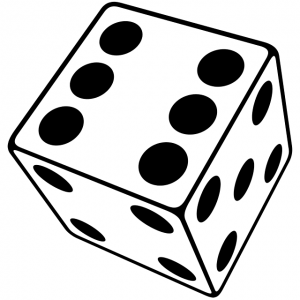Learn the 4 Things You Must Know Before Taking the GMAT!   Download it Now!

# Pay Attention to Mutually Exclusive EventsPretty much all probability questions on the GMAT can be answered using three core rules. We teach them in detail in our Probability and Combinatorics course, but I want to take a second to tease out an important nuance to the Addition Rule (officially known as the “Union” rule) here.

### The Addition Rule of Probability

The Addition Rule refers to situations where you’re trying to find the probability of something OR something else.

To keep things simple, we teach that whenever you see the word “or” in GMAT probably questions, you simply add the two independent probabilities.

For example:

If you roll one standard six-sided die, what is the probability of rolling either a 2 or a 3?

The answer is pretty straightforward. The probability of rolling a 2 or a 3 equals the probability of rolling a 2 PLUS the probability of rolling a 3. The notation you can use on your scratch paper is:

### p(rolling a 2 or 3) = p(rolling a 2) + p(rolling a 3)

(Note: the “p” stands for “probability”)

The independent probability of rolling a 2 is 1/6 because there’s only one 2 out of 6 possible outcomes (i.e. sides of the die). Likewise, the independent probability of rolling a 3 is also 1/6. So the probability of rolling a 2 or a 3 = 1/6 + 1/6 = 2/6 = 1/3.

Easy, right?

But be careful…

### The Expanded Addition Rule and Mutual Exclusivity

While this simplified Addition Rule will get the job done for you on a large number of GMAT probability questions, technically what we’ve done so far only applies if the two events are mutually exclusive. Mutually exclusive means that the two events can’t happen at the same time. It’s impossible, for example, to roll a 2 and a 3 at the same time on only one roll of a die. Rolling a 2 is mutually exclusive to rolling a 3. Thus, we simply add those two independent probabilities when finding the probability of rolling either.

However, sometimes — especially on harder GMAT probability questions — the two events are not mutually exclusive. And we need to account for that.

So, it’s worth adding an important nuance to the Addition Rule. Specifically, the full Addition Rule for probability is as follows:

### p(A or B) = p(A) + p(B) – p(A and B)

Notice that whenever the two events A and B are not mutually exclusive, i.e. they could possibly exist together, you need to subtract out the probability of that occurring. And that makes sense, right? We don’t want to include that overlap.

### Wording Tip + Application Example

In fact, you should get in the habit of mentally adding some extra wording to these types of GMAT probability questions to remind yourself to account for non-mutually exclusive events, if necessary.

That wording is: “…but not both.”

Consider our earlier example. The question itself reads, What is the probability of rolling either a 2 or a 3? But what’s implied is, What is the probability of rolling either a 2 or a 3 but not both?

Now obviously in the case of rolling a 2 or 3 on one roll of a normal die, it’s not possible to roll both at the same time. So that extra wording isn’t relevant.

But sometimes it is.

Take a look at the following example that illustrates this point:

A card is drawn randomly from a deck of 52 ordinary playing cards. You win \$10 if the card is a spade or an ace. What is the probability that you will win the \$10?

(A) 1/13
(B) 13/52
(C) 4/13
(D) 17/52
(E) None of the above

You could change up the wording of the question to read, What is the probability of drawing either a spade or an ace? since that’s how you win the \$10. And of course we now know to mentally add the following words as well: What is the probability of drawing either a space or an ace, but not both?

Do you see how this question differs from the earlier one about rolling a 2 or a 3?

This one is different because it is possible to pick a card that is both an Ace and a Spade (i.e. the Ace of Spades). There’s one such card in a standard 52-card deck, and so we have to subtract out the probability of drawing that card.

Therefore, based on the full Addition Rule:

p(Spade or Ace) = p(Spade) + p(Ace) – p(Spade and Ace)

p(Spade or Ace) = 13/52 + 4/52 – 1/52

p(Spade or Ace) = 16/52 = 4/13